# 前言

bert模型是谷歌2018年10月底公布的，反响巨大，效果不错，在各大比赛上面出类拔萃，它的提出主要是针对word2vec等模型的不足，在之前的预训练模型（包括word2vec，ELMo等）都会生成词向量，这种类别的预训练模型属于domain transfer。而近一两年提出的ULMFiT，GPT，BERT等都属于模型迁移，说白了BERT 模型是将预训练模型和下游任务模型结合在一起的，核心目的就是：是把下游具体NLP任务的活逐渐移到预训练产生词向量上。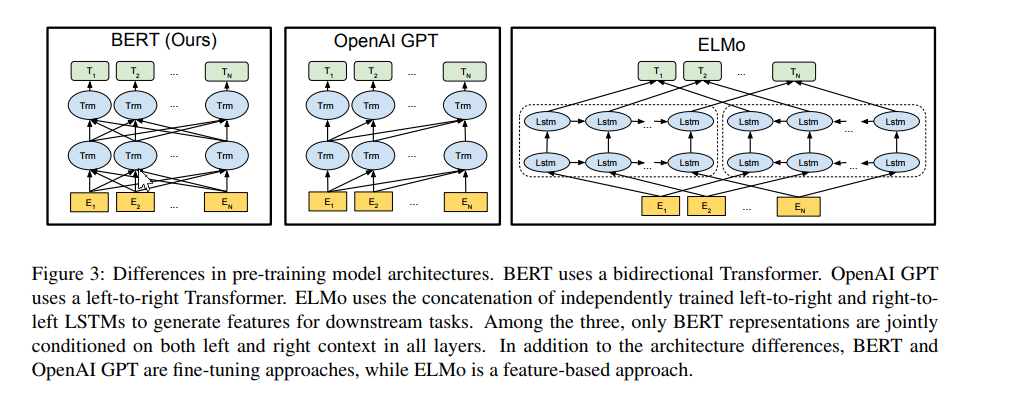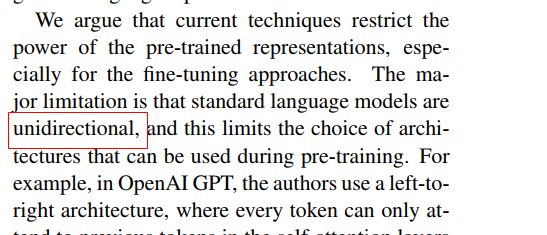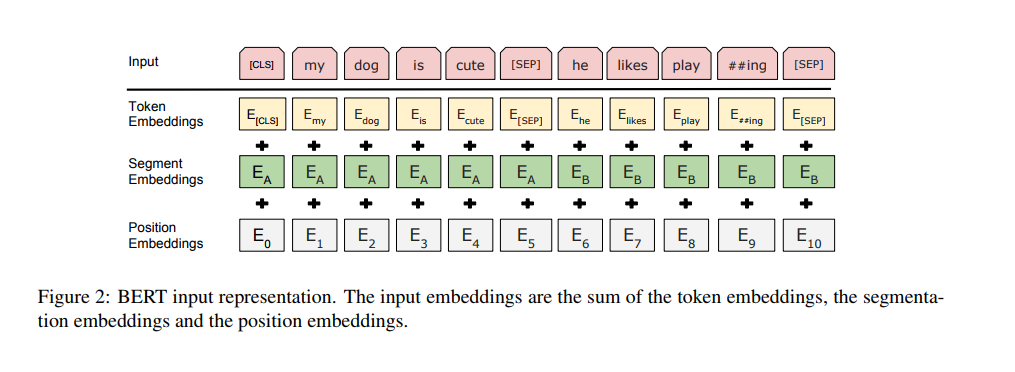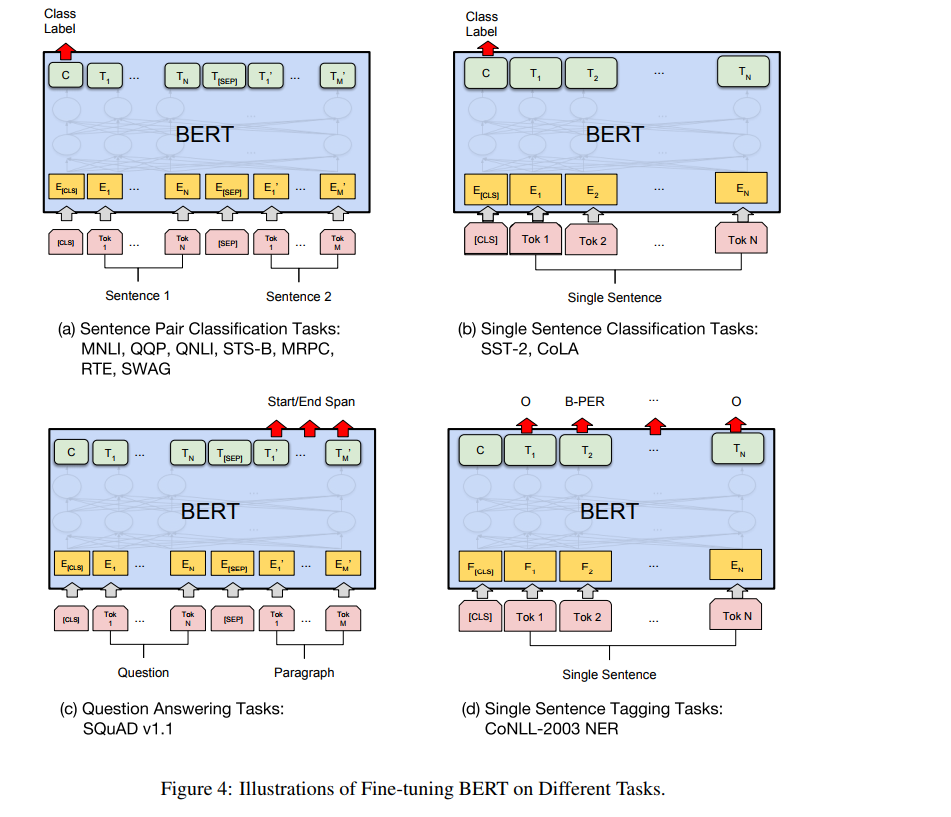https://github.com/yuanxiaosc/Entity-Relation-Extraction

# 解读

## run_classifier.py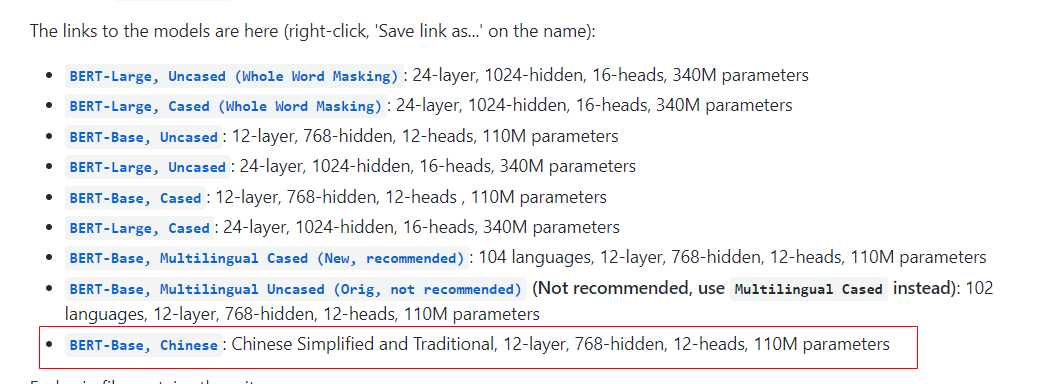### run_classifier.py中的类如下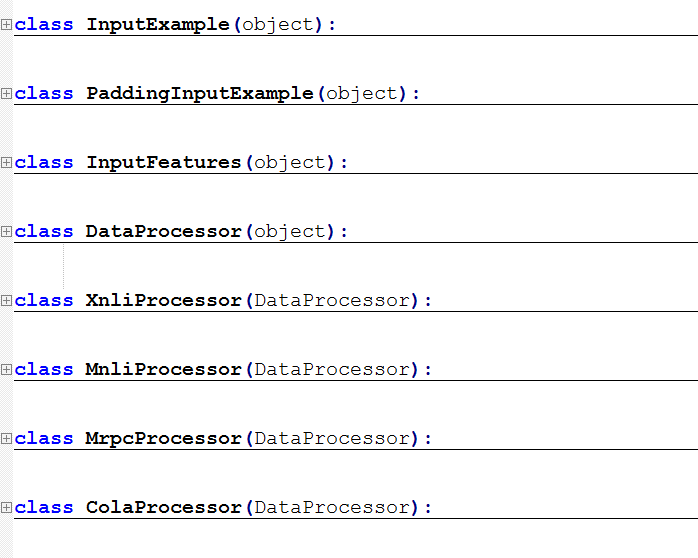InputExample类主要定义了一些数据预处理后要生成的字段名，如下：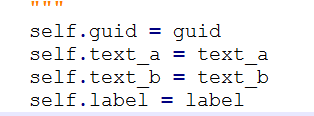guid就是一个id号，一般将数据处理成train、dev、test数据集，那么这里定义方式就可以是相应的数据集+行号（句子）

text_a 就是当前的句子，text_b是另一个句子，因为有的任务需要两个两个句子，如果任务中没有的话，可以将text_b设为None

label就是标签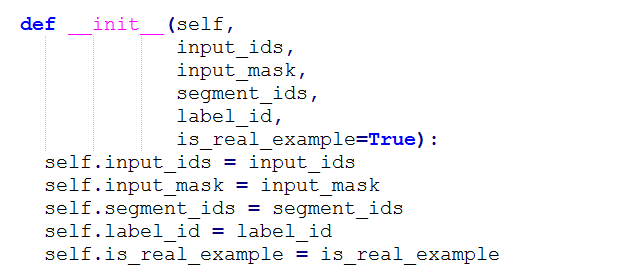DataProcessor，这是一个数据预处理的基类，里面定义了一些基本方法

XnliProcessor、MnliProcessor、MrpcProcessor、ColaProcessor四个类是对DataProcessor的具体实现，这里之所以列举了四个是尽可能多的给用户呈现出各种demo，具体到实际使用的时候我们只需要参考其写法，定义一个自己的数据预处理类即可，其中一般包括如下几个方法：get_train_examples，get_dev_examples，get_test_examples，get_labels，_create_examples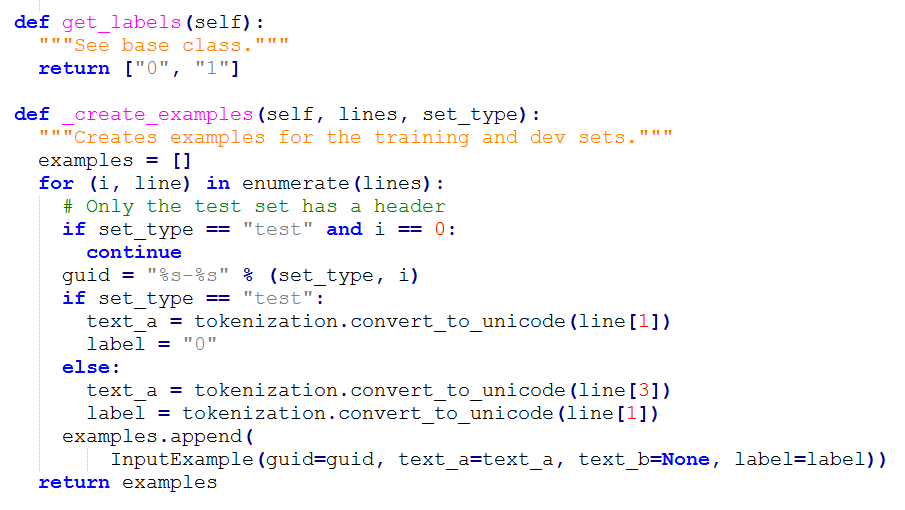### convert_single_example：

bert的输入：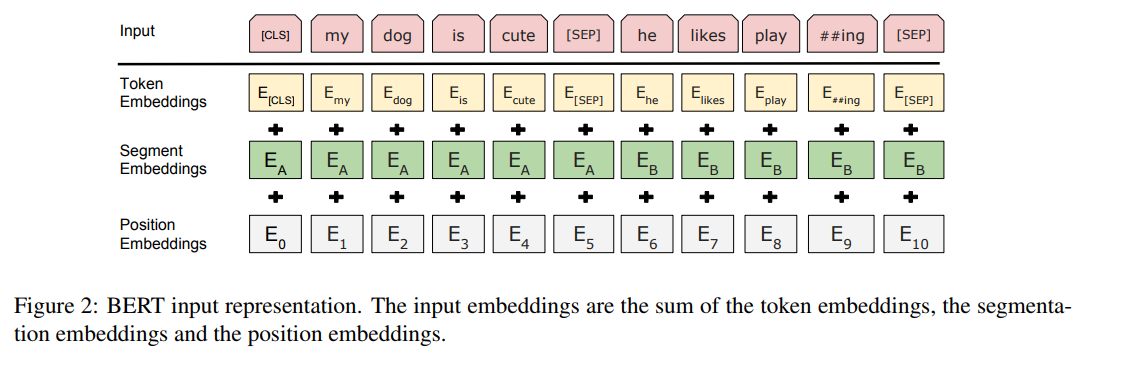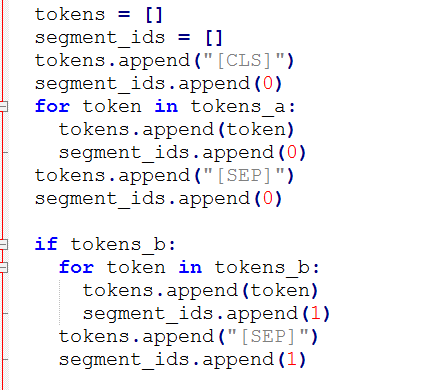input_ids中就是记录的是使用FullTokenizer类convert_tokens_to_ids方法将tokens转化成单个字的id

segment_ids就是句子级别（上下句）的标签，大概形式：

# (a) For sequence pairs:
#  tokens:   [CLS] is this jack ##son ##ville ? [SEP] no it is not . [SEP]
#  type_ids: 0     0  0    0    0     0       0 0     1  1  1  1   1 1
# (b) For single sequences:
#  tokens:   [CLS] the dog is hairy . [SEP]
#  type_ids: 0     0   0   0  0     0 0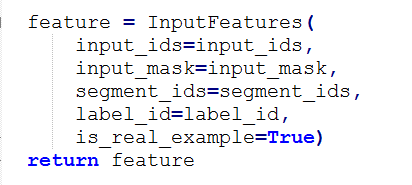### truncate_seq_pair

-----------------------------------------------------------------------------------------------------------------------------------------------------------------------

### model_fn_builder

model_fn_builder是壳，create_model是核心，其内部定义了loss,预测概率以及预测结果等等。

### model_fn_builder

https://www.cnblogs.com/jiangxinyang/p/10341392.html

### create_model

##############################################重中之重############################################

##############################################重中之重############################################

##############################################重中之重############################################

def create_model(bert_config, is_training, input_ids, input_mask, segment_ids,                 labels, num_labels, use_one_hot_embeddings):  """Creates a classification model."""  model = modeling.BertModel(      config=bert_config,      is_training=is_training,      input_ids=input_ids,      input_mask=input_mask,      token_type_ids=segment_ids,      use_one_hot_embeddings=use_one_hot_embeddings)   # In the demo, we are doing a simple classification task on the entire  # segment.  #  # If you want to use the token-level output, use model.get_sequence_output()  # instead.  output_layer = model.get_pooled_output()   hidden_size = output_layer.shape[-1].value   output_weights = tf.get_variable(      "output_weights", [num_labels, hidden_size],      initializer=tf.truncated_normal_initializer(stddev=0.02))   output_bias = tf.get_variable(      "output_bias", [num_labels], initializer=tf.zeros_initializer())   with tf.variable_scope("loss"):    if is_training:      # I.e., 0.1 dropout      output_layer = tf.nn.dropout(output_layer, keep_prob=0.9)     logits = tf.matmul(output_layer, output_weights, transpose_b=True)    logits = tf.nn.bias_add(logits, output_bias)    probabilities = tf.nn.softmax(logits, axis=-1)    log_probs = tf.nn.log_softmax(logits, axis=-1)     one_hot_labels = tf.one_hot(labels, depth=num_labels, dtype=tf.float32)     per_example_loss = -tf.reduce_sum(one_hot_labels * log_probs, axis=-1)    loss = tf.reduce_mean(per_example_loss)     return (loss, per_example_loss, logits, probabilities)

model = modeling.BertModel(      config=bert_config,      is_training=is_training,      input_ids=input_ids,      input_mask=input_mask,      token_type_ids=segment_ids,      use_one_hot_embeddings=use_one_hot_embeddings

config是bert的配置文件，在开头下载的中文模型中里面有，直接加载即可

use_one_hot_embeddings是根据是不是用GPU而定的，其他字段上述都说过啦

bert模型的输出：

model.get_sequence_output()model.get_pooled_output()

output_weights和output_bias就是对应全连接成的权值，后面就是loss,使用了tf.nn.log_softmax应该是一个多分类，多标签的话可以使用tf.nn.sigmoid，比较简单就不再说啦

### main

processors = {      "cola": ColaProcessor,      "mnli": MnliProcessor,      "mrpc": MrpcProcessor,      "xnli": XnliProcessor,  }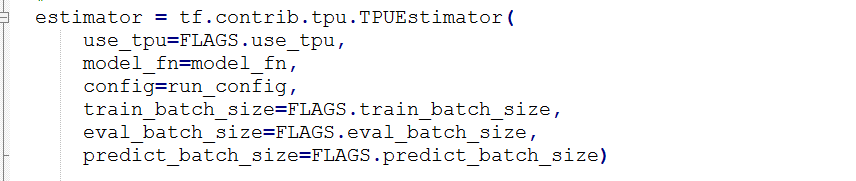## 总结：

1） 继承DataProcessor定义一个自己的数据预处理类

2） 在create_model中定义自己的具体下游工作

# 实践

## 前言

https://github.com/yuanxiaosc/Entity-Relation-Extraction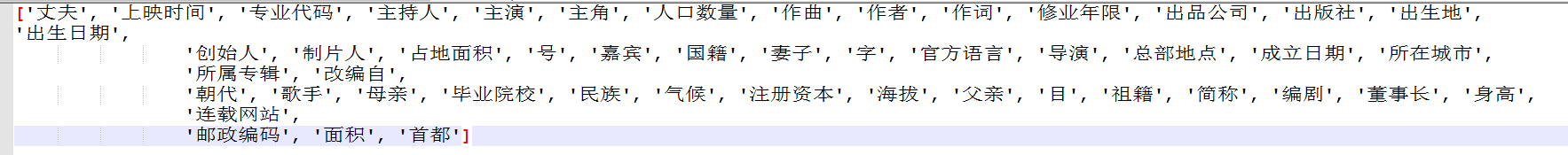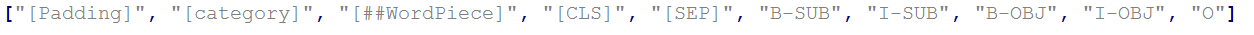SUB对应的就是subject，B-SUB就是第一个实体开始的位置，后续的是I-SUB

OBJ就是第二个实体

## 总体简介

### run_predicate_classification.py， run_sequnce_labeling.py

produce_submit_json_file.py 就是将结果转化成比赛需要提交的数据格式，注意到最后的格式还需要实体对的类型，我们通过上面得到了三元组，但是还缺实体的类型，代码中是使用了字典，依据关系确定对应实体类型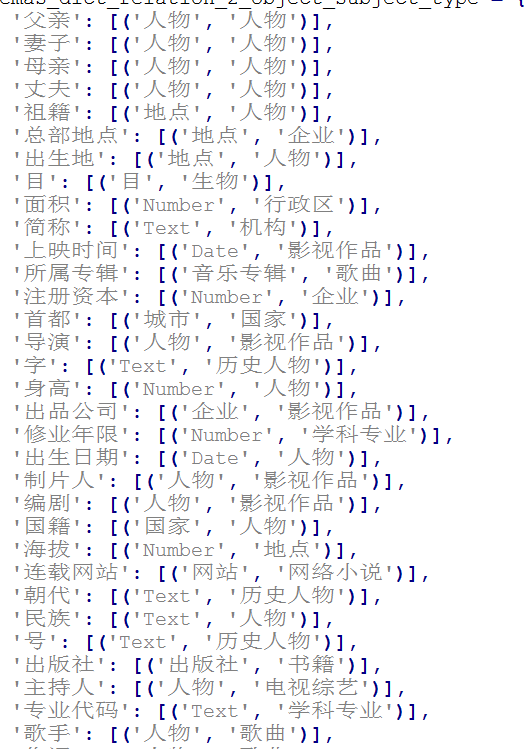### bin

evaluation文件夹是评价函数

predicate_classifiction和subject_object_labeling是对应的第一个模型和第二个模型进行前需要的数据预处理脚本

## 预测阶段

### 准备关系分类数据

python bin/predicate_classifiction/predicate_data_manager.py

train和dev两个json文件大体样式是：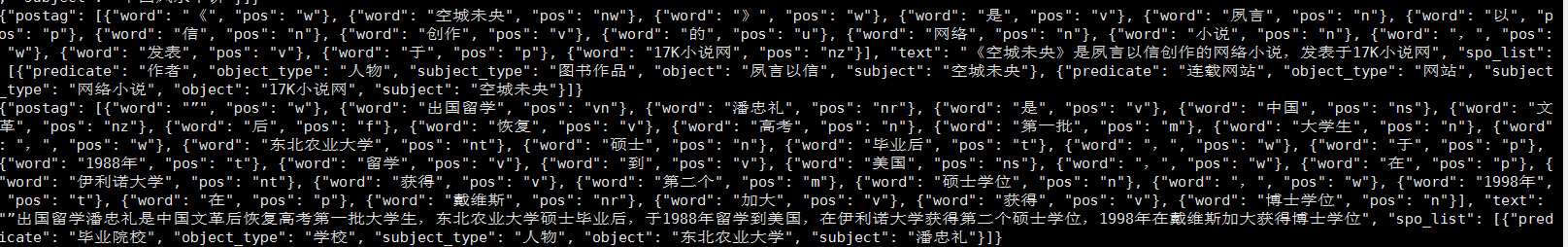test.json: 没有spo_list,待预测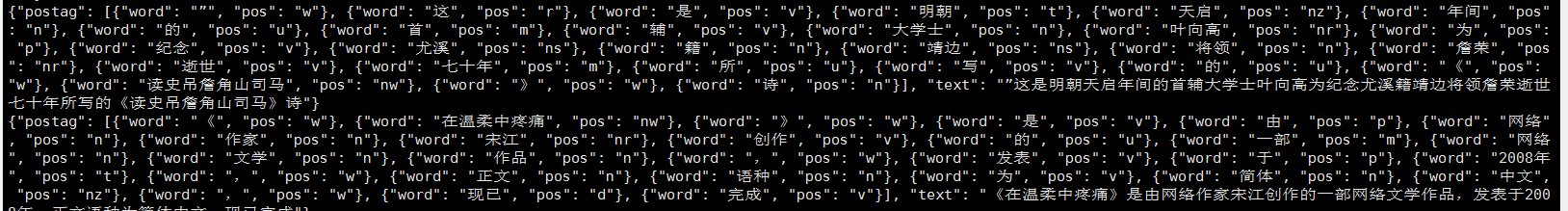predicate_out.txt  ：提取出每一个句子的关系集合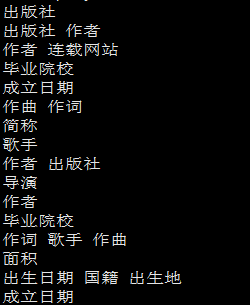text.txt  ：每一个句子的文本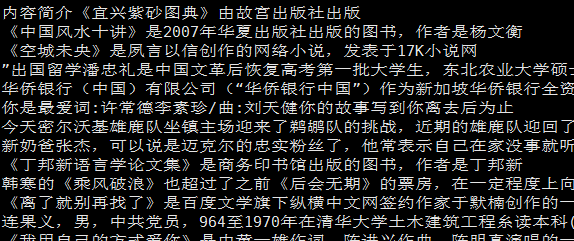token_in_not_UNK.txt ：分字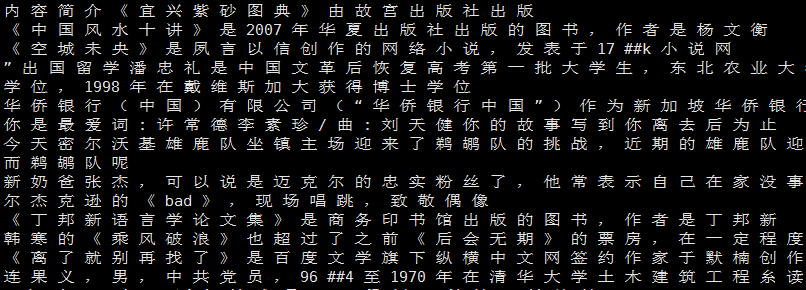token_in.txt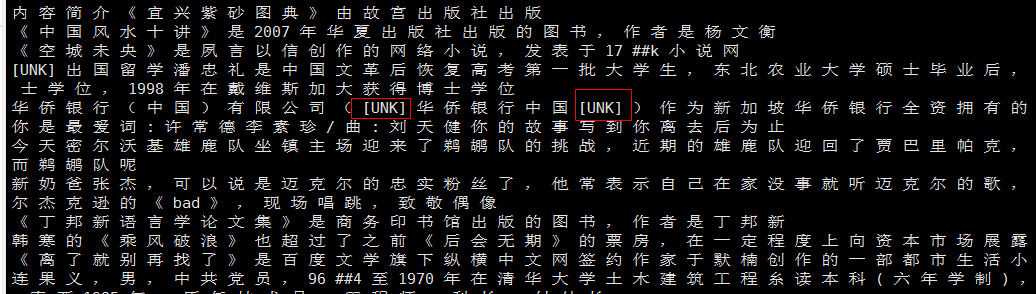predicate_data_manager.py set: Competition_Mode = False

### 关系分类模型训练

python run_predicate_classification.py \--task_name=SKE_2019 \--do_train=true \--do_eval=false \--data_dir=bin/predicate_classifiction/classification_data \--vocab_file=pretrained_model/chinese_L-12_H-768_A-12/vocab.txt \--bert_config_file=pretrained_model/chinese_L-12_H-768_A-12/bert_config.json \--init_checkpoint=pretrained_model/chinese_L-12_H-768_A-12/bert_model.ckpt \--max_seq_length=128 \--train_batch_size=32 \--learning_rate=2e-5 \--num_train_epochs=6.0 \--output_dir=./output/predicate_classification_model/epochs6/

SKE_2019_Multi_Label_Classification_Processor

 with tf.variable_scope("loss"):        if is_training:            # I.e., 0.1 dropout            output_layer = tf.nn.dropout(output_layer, keep_prob=0.9)         logits_wx = tf.matmul(output_layer, output_weights, transpose_b=True)        logits = tf.nn.bias_add(logits_wx, output_bias)        probabilities = tf.sigmoid(logits)        label_ids = tf.cast(labels, tf.float32)        per_example_loss = tf.reduce_sum(            tf.nn.sigmoid_cross_entropy_with_logits(logits=logits, labels=label_ids), axis=-1)        loss = tf.reduce_mean(per_example_loss)         return loss, per_example_loss, logits, probabilities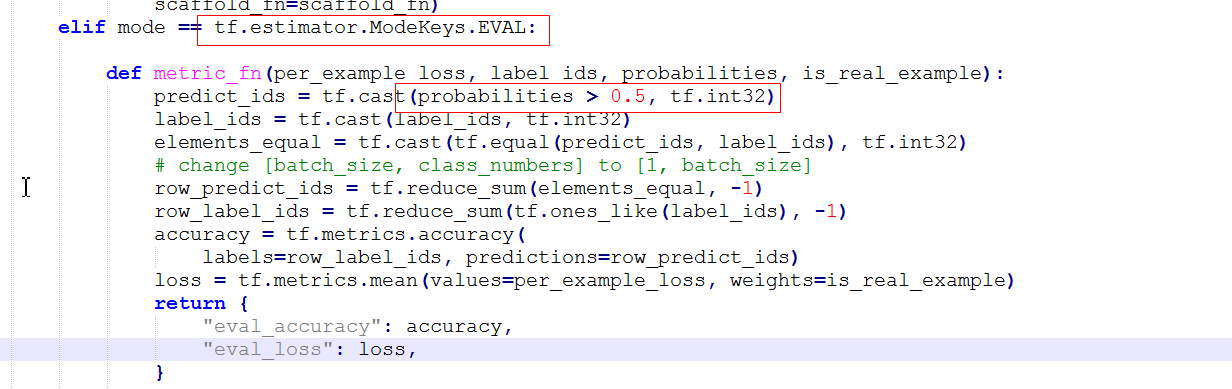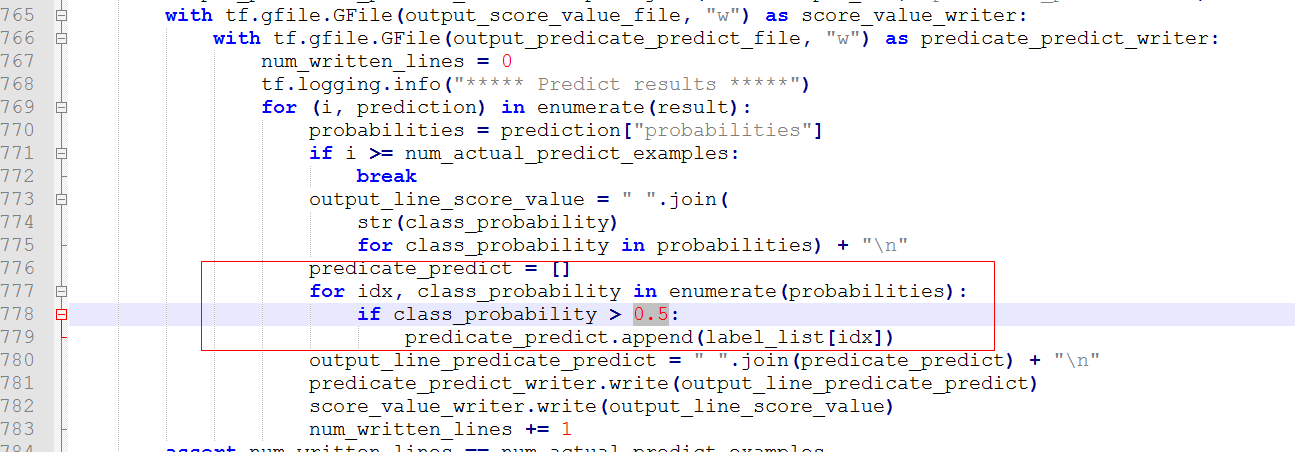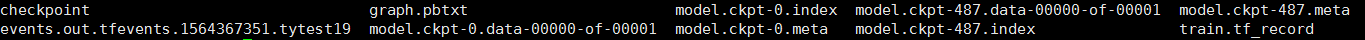### 准备序列标注数据

python bin/subject_object_labeling/sequence_labeling_data_manager.py

bert_tokener_error_log.txt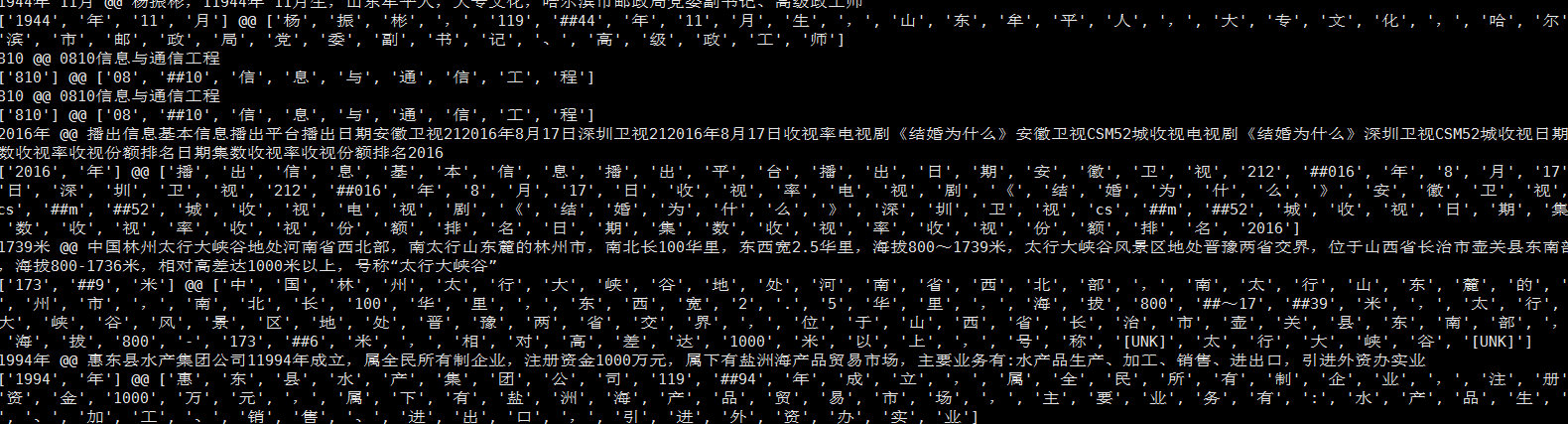text.txt：可以看到如果句子有多个三元组是分成多个样本的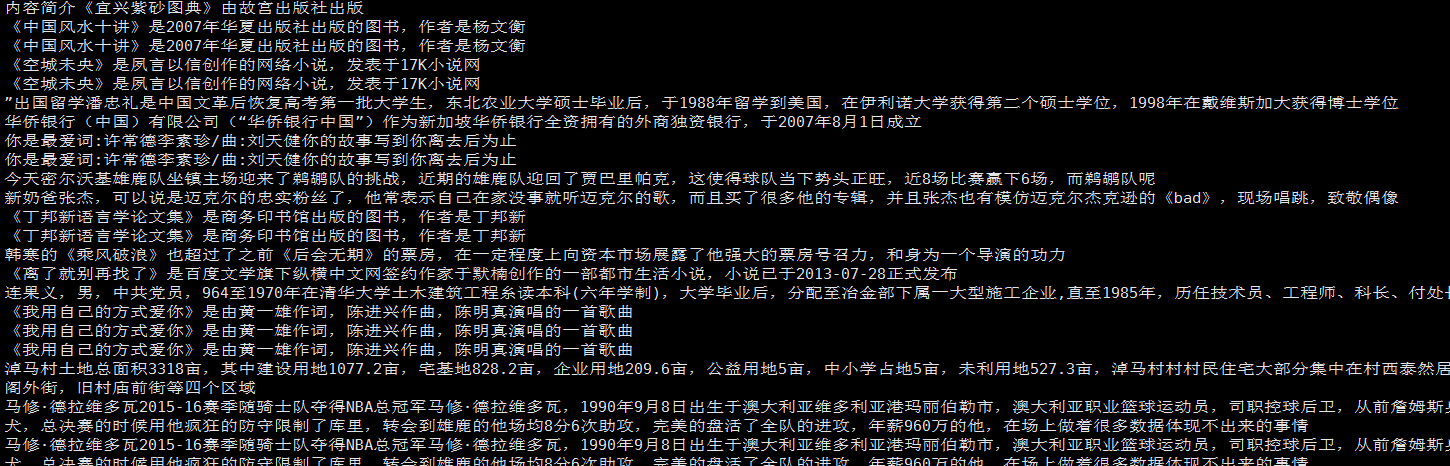token_in_not_UNK.txt  token_in.txt和前面类似

token_label_and_one_prdicate_out.txt：可以看到SUB和OBJ就是对应实体对的标示，后面是关系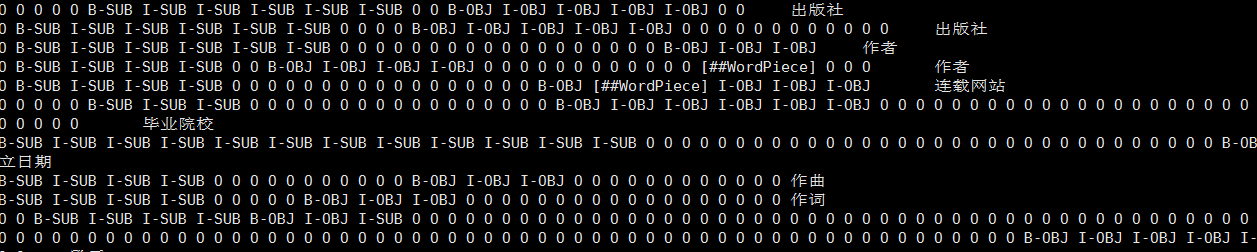### 序列标注模型训练

python run_sequnce_labeling.py \--task_name=SKE_2019 \--do_train=true \--do_eval=false \--data_dir=bin/subject_object_labeling/sequence_labeling_data \--vocab_file=pretrained_model/chinese_L-12_H-768_A-12/vocab.txt \--bert_config_file=pretrained_model/chinese_L-12_H-768_A-12/bert_config.json \--init_checkpoint=pretrained_model/chinese_L-12_H-768_A-12/bert_model.ckpt \--max_seq_length=128 \--train_batch_size=32 \--learning_rate=2e-5 \--num_train_epochs=9.0 \--output_dir=./output/sequnce_labeling_model/epochs9/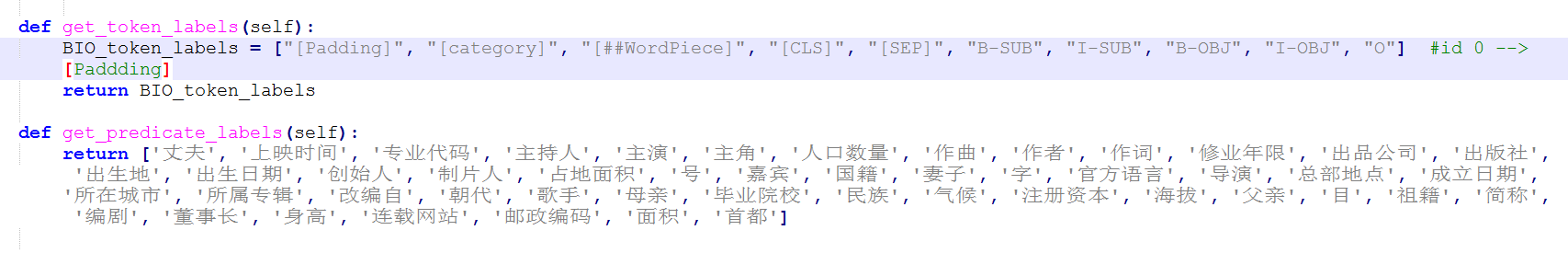predicate_output_layer = model.get_pooled_output()     intent_hidden_size = predicate_output_layer.shape[-1].value     predicate_output_weights = tf.get_variable(        "predicate_output_weights", [num_predicate_labels, intent_hidden_size],        initializer=tf.truncated_normal_initializer(stddev=0.02))     predicate_output_bias = tf.get_variable(        "predicate_output_bias", [num_predicate_labels], initializer=tf.zeros_initializer())     with tf.variable_scope("predicate_loss"):        if is_training:            # I.e., 0.1 dropout            predicate_output_layer = tf.nn.dropout(predicate_output_layer, keep_prob=0.9)         predicate_logits = tf.matmul(predicate_output_layer, predicate_output_weights, transpose_b=True)        predicate_logits = tf.nn.bias_add(predicate_logits, predicate_output_bias)        predicate_probabilities = tf.nn.softmax(predicate_logits, axis=-1)        predicate_prediction = tf.argmax(predicate_probabilities, axis=-1, output_type=tf.int32)        predicate_labels = tf.one_hot(predicate_label_id, depth=num_predicate_labels, dtype=tf.float32)        predicate_per_example_loss = tf.reduce_sum(tf.nn.sigmoid_cross_entropy_with_logits(logits=predicate_logits, labels=predicate_labels), -1)        predicate_loss = tf.reduce_mean(predicate_per_example_loss)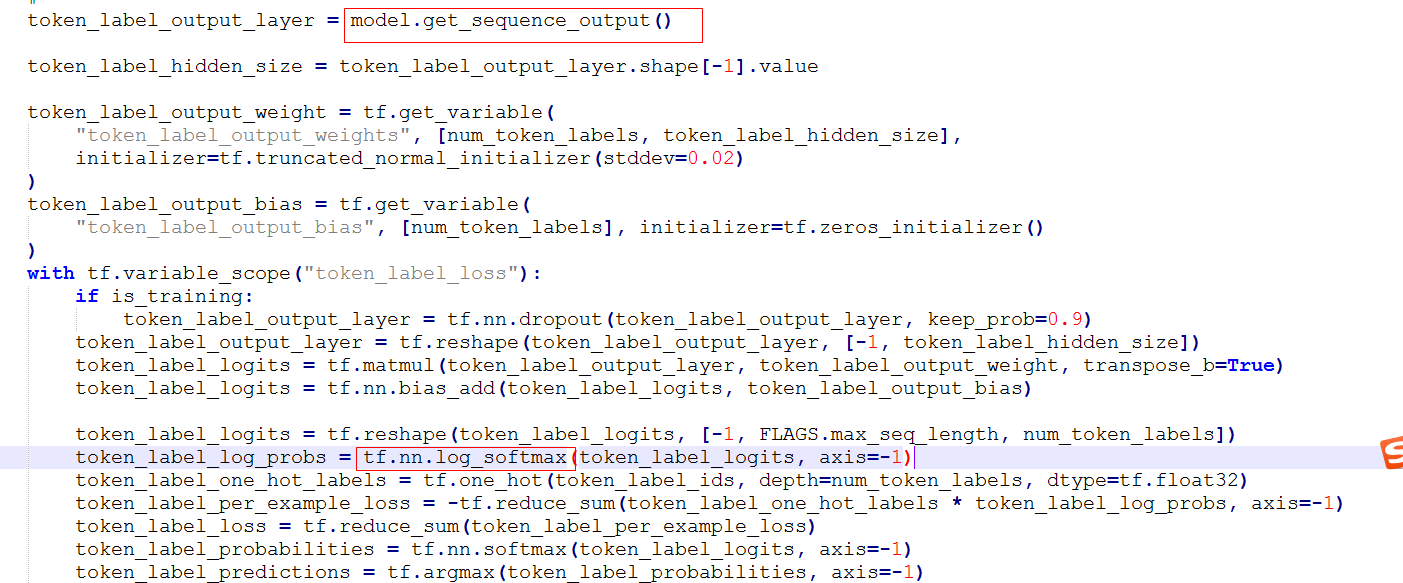loss = 0.5 * predicate_loss + token_label_loss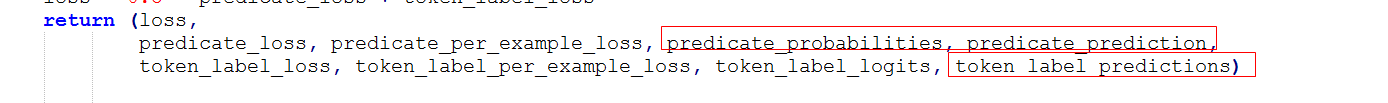##########################################训练过程就结束啦##########################################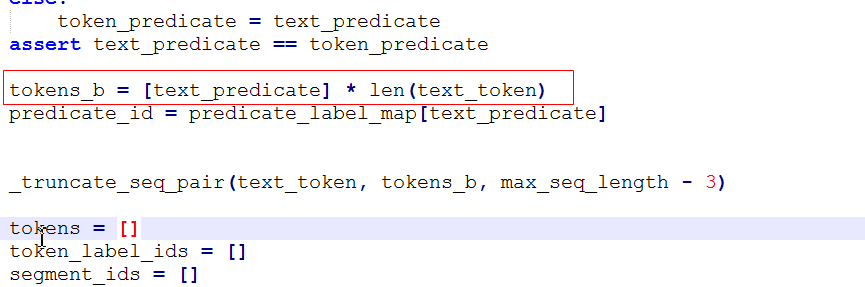tokens     :           CLS   胡   歌   是   仙   剑   的   主   演   SEP   主演   主演   主演   主演   主演   主演   主演   主演  SEP

input_ids :           101   200 201 202 203 204 205 206 207 102   2  2   2  2  2   2  2   2   102  0 0 0

segment_ids :       0       0     0     0    0     0    0     0     0     0        1       1        1         1         1         1      1         1       1 0 0 0

input_mask :     1   1   1   1   1    1    1     1     1     1    1       1        1         1         1         1      1         1       1  0 0 0

token_label   :        [CLS]    B-SUB I-SUB o B-OBJ I-OBJ o o o [SEP] [category] [category] [category] [category] [category]

predicate_id : 1

这里是将所有类别制作成一个字典，类如主演的value就是1，上述之所以是2，是因为代码中同一加了一个偏置

那么这里类别的id和经过tokenizer.convert_tokens_to_ids转化后的字id有没有可能是冲突的呢？没有！，因为

tokenizer.convert_tokens_to_ids没有用1-100之内的id，当前类别又没有超过100，所以不会冲突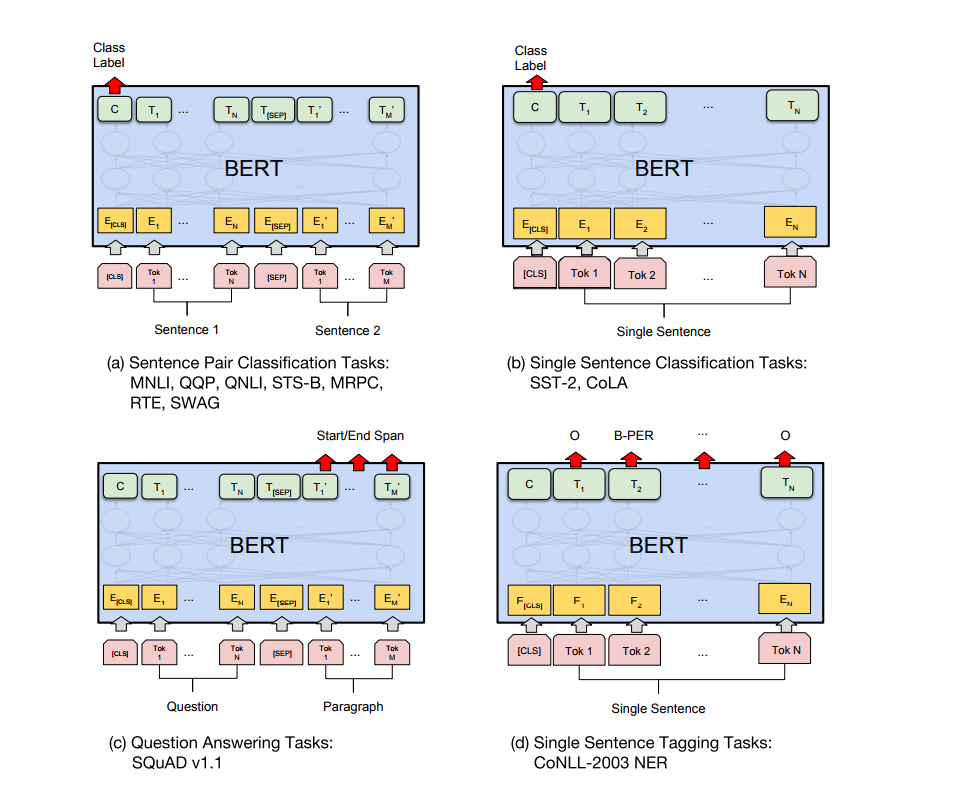##################################################################################################

## 预测阶段

### 关系分类模型预测

python run_predicate_classification.py \  --task_name=SKE_2019 \  --do_predict=true \  --data_dir=bin/predicate_classifiction/classification_data \  --vocab_file=pretrained_model/chinese_L-12_H-768_A-12/vocab.txt \  --bert_config_file=pretrained_model/chinese_L-12_H-768_A-12/bert_config.json \  --init_checkpoint=output/predicate_classification_model/epochs6/model.ckpt-478 \  --max_seq_length=128 \  --output_dir=./output/predicate_infer_out/epochs6/ckpt478

init_checkpoint

predicate_predict.txt类别预测结果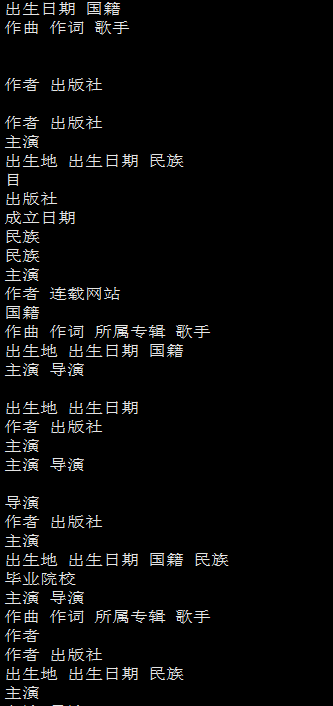predicate_score_value.txt：类别概率，以0.5为阈值确定最终结果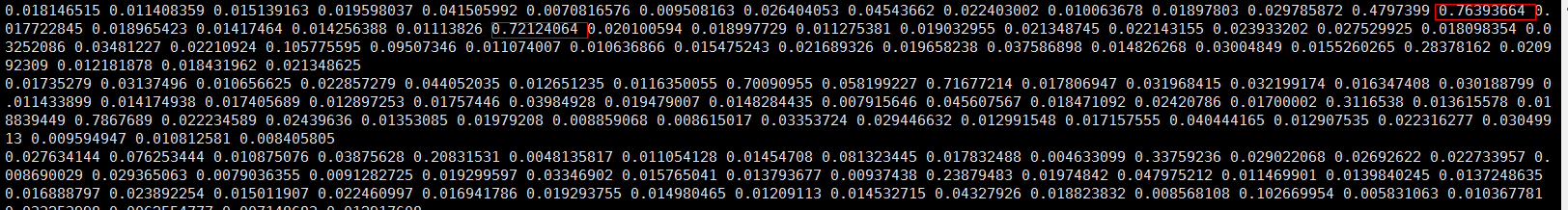## 把关系分类模型预测结果转换成序列标注模型的预测输入

python bin/predicate_classifiction/prepare_data_for_labeling_infer.py

text_and_one_predicate.txt：单句文本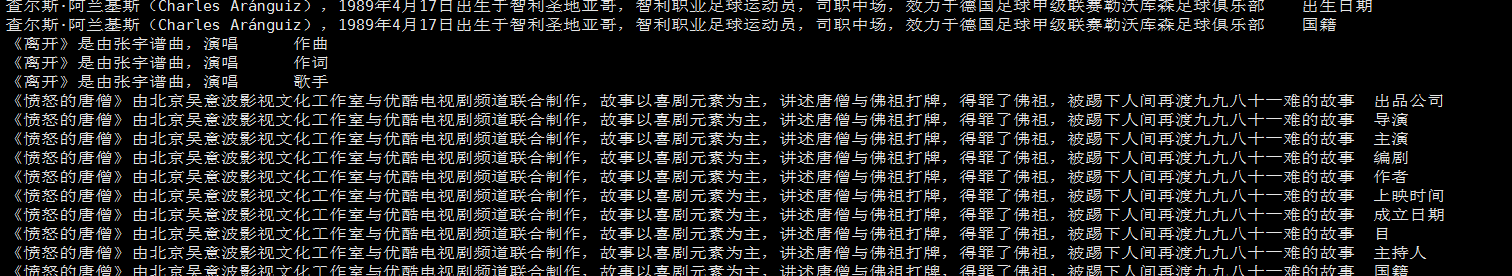token_in_and_one_predicate.txt：单句文本以及对应的关系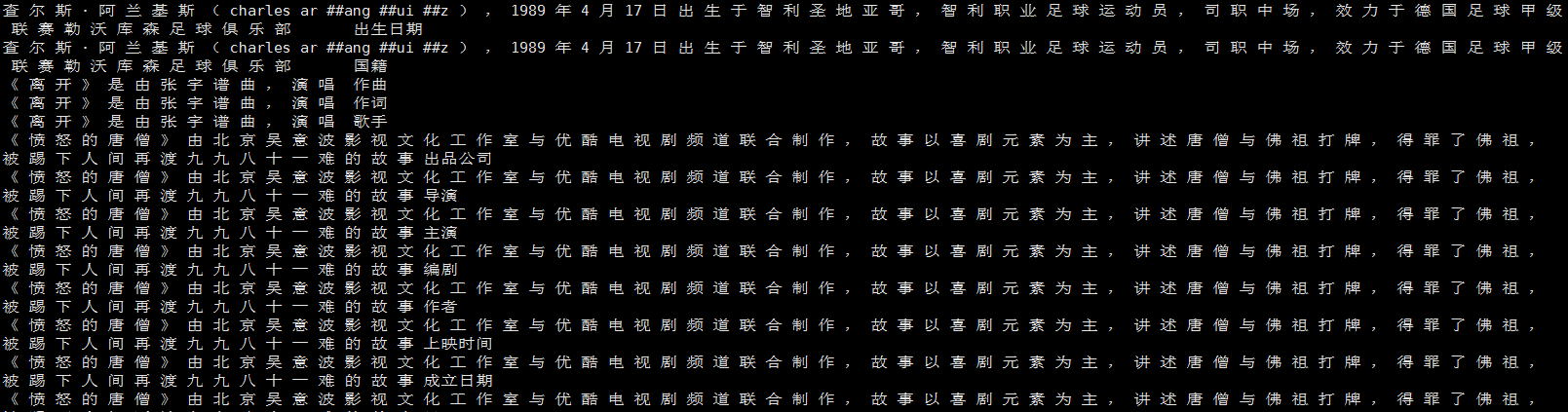token_in_not_UNK_and_one_predicate.txt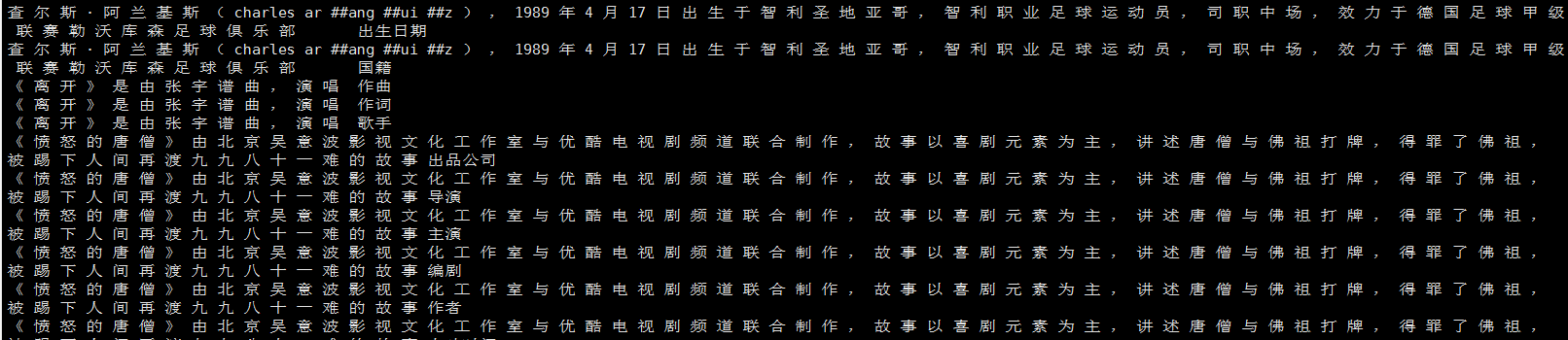### 序列标注模型预测

python run_sequnce_labeling.py \  --task_name=SKE_2019 \  --do_predict=true \  --data_dir=bin/subject_object_labeling/sequence_labeling_data \  --vocab_file=pretrained_model/chinese_L-12_H-768_A-12/vocab.txt \  --bert_config_file=pretrained_model/chinese_L-12_H-768_A-12/bert_config.json \  --init_checkpoint=output/sequnce_labeling_model/epochs9/model.ckpt-1237 \  --max_seq_length=128 \  --output_dir=./output/sequnce_infer_out/epochs9/ckpt1237

predicate_predict.txt：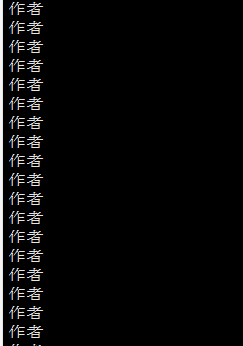predicate_probabilities.txt：关系预测概率，单标签，选取最大的概率作为最终结果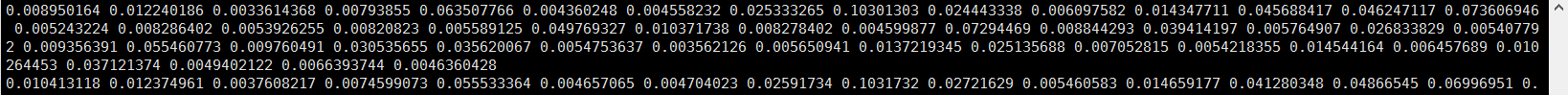token_label_predictions.txt：实体表示预测结果（其实对应每一个token也是单标签多分类）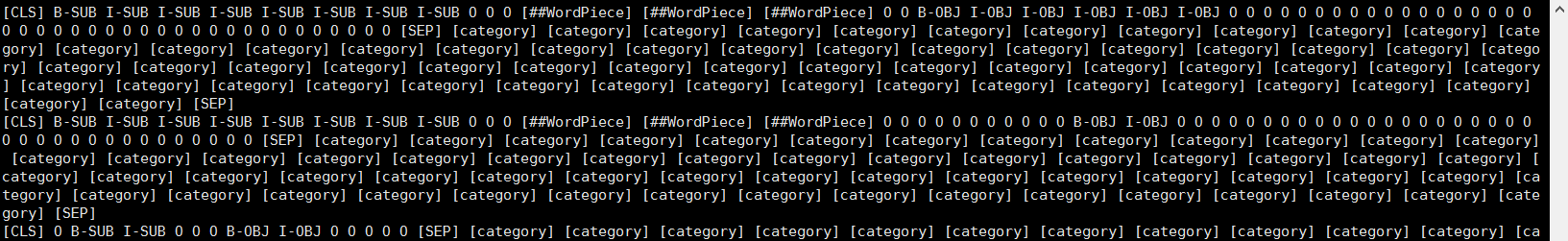### 生成实体-关系结果

python produce_submit_json_file.py

keep_empty_spo_list_subject_predicate_object_predict_output.json：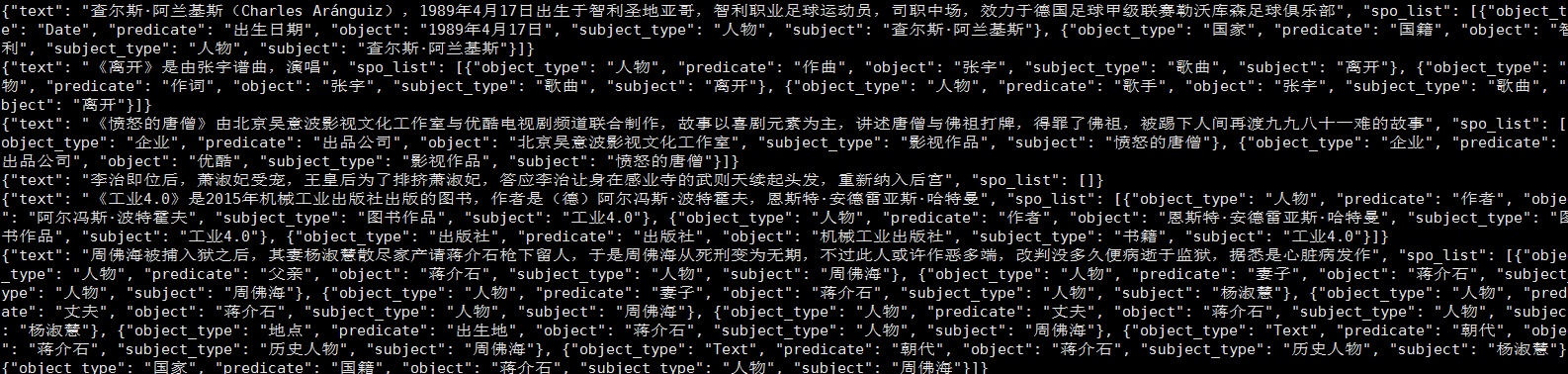## 评价

### 关系抽取模型性能

python bin/evaluation/evaluate_classifiction.py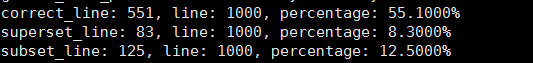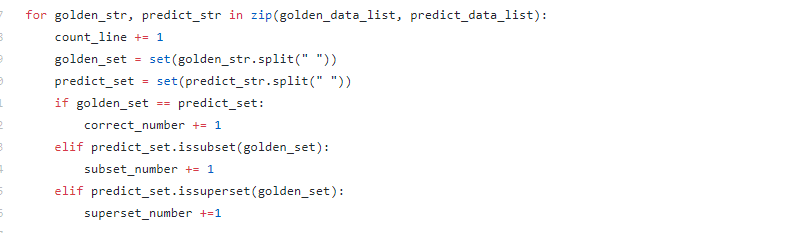### 实体抽取模型性能

python bin/evaluation/evaluate_labeling.py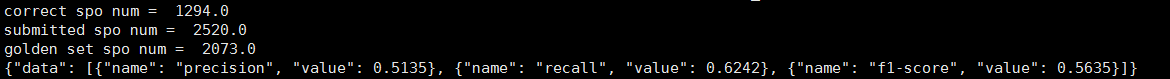# 总结

bert的输出：model.get_sequence_output() model.get_pooled_output()

写过一篇博客https://blog.csdn.net/weixin_42001089/article/details/95493249）都是先进行实体抽取，后进行关系抽取，

如果想要既使用后面的顺序（因为有的场景是给定句子和一个实体对来抽取判断关系）又想利用bert进行相关工作，那么

借鉴这里的思路，也是两个模型，第一个模型没问题就是使用实体标示的样本去训练，就是一个单标签多分类，那么第二

在进行第二个模型的时候可能要想一下，即输入怎么将当前实体对这一信息输入进去，有想法的可以试一下

©️2019 CSDN 皮肤主题: 深蓝海洋 设计师: CSDN官方博客